# Optimal control problem for stationary quasi-optic equations

## Abstract

In this paper, an optimal control problem was taken up for a stationary equation of quasi optic. For the stationary equation of quasi optic, at first judgment relating to the existence and uniqueness of a boundary value problem was given. By using this judgment, the existence and uniqueness of the optimal control problem solutions were proved. Then we state a necessary condition to an optimal solution. We proved differentiability of a functional and obtained a formula for its gradient. By using this formula, the necessary condition for solvability of the problem is stated as the variational principle.

## 1 Introduction

Optimal control theory for the quantum mechanic systems described with the Schrödinger equation is one of the important areas of modern optimal control theory. Actually, a stationary quasi-optics equation is a form of the Schrödinger equation with complex potential. Such problems were investigated in . Optimal control problem for nonstationary Schrödinger equation of quasi optics was investigated for the first time in .

## 2 Formulation of the problem

We are interested in finding the problem of the minimum of the functional

${J}_{\alpha }\left(v\right)={\parallel \psi \left(\mathbf{\cdot },L\right)-y\parallel }_{{L}_{2}\left(0,l\right)}^{2}+\alpha {\parallel v-\omega \parallel }_{H}^{2}$
(1)

in the set

$\begin{array}{rcl}V& \equiv & \left\{v=\left({v}_{0},{v}_{1},{\phi }_{0},{\phi }_{1}\right),{v}_{m}\in {L}_{2}\left(0,l\right),{v}_{1}\left(z\right)\ge 0,\stackrel{0}{\mathrm{\forall }}z\in \left(0,L\right),\\ {\parallel {v}_{m}\parallel }_{{L}_{2}\left(0,l\right)}\le {b}_{m},{\phi }_{m}\in {L}_{2}\left(0,l\right),{\parallel {\phi }_{m}\parallel }_{{L}_{2}\left(0,l\right)}\le {d}_{m},m=0,1\right\}\end{array}$

under the condition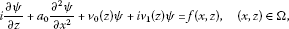(2)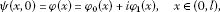(3)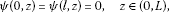(4)

where $i=\sqrt{-1}$, ${a}_{0}>0$, $l>0$, $L>0$, $\alpha \ge 0$, ${b}_{0}\ge 0$, ${b}_{1}>0$, ${d}_{0}>0$, ${d}_{1}>0$ are numbers, $x\in \left[0,l\right]$, $z\in \left[0,L\right]$, ${\mathrm{\Omega }}_{z}=\left(0,l\right)×\left(0,z\right)$, $\mathrm{\Omega }={\mathrm{\Omega }}_{\mathrm{L}}$, $y\left(x\right)$, $f\left(x,z\right)$ are complex valued measurable functions and satisfy the conditions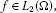(5)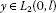(6)

respectively, $\omega =\left({\omega }_{0},{\omega }_{1},{\varpi }_{0},{\varpi }_{1}\right)$ and $H={\left({L}_{2}\left(0,l\right)\right)}^{2}×{\left({L}_{2}\left(0,L\right)\right)}^{2}$. ${L}_{2}\left(0,l\right)$ is a Hilbert space that consists of all functions in $\left(0,l\right)$, which are measurable and square-integrable. ${L}_{2}\left(\mathrm{\Omega }\right)$ is the well-known Lebesgue space consisting of all functions in Ω, which are measurable and square-integrable.

The problem of finding a function $\psi =\psi \left(x,\mathrm{z}\right)\equiv \psi \left(x,z;v\right)$ under the condition (2)-(4) for each $\mathrm{\forall }v\in V$, which is a boundary value problem, is a function for Eq. (2).

Generalized solution of this problem is a function $\psi =\psi \left(x,\mathrm{z}\right)\equiv \psi \left(x,z;v\right)$ belonging to the ${C}^{0}\left(\left[0,L\right],{L}_{2}\left(0,l\right)\right)$, and it satisfies the integral identity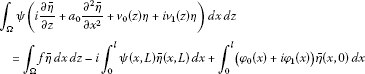(7)

for $\mathrm{\forall }\eta \in {C}^{0}\left(\left[0,L\right],{L}_{2}\left(0,l\right)\right)$.

## 3 Existence and uniqueness of a solution of the optimal control problem

In this section, we prove the optimal control problem using the Galerkin method and the existence and uniqueness of a solution of the problem (1)-(4).

Theorem 1 Suppose that a function f satisfies the condition (5). So, for each $\mathrm{\forall }v\in V$, the problem (2)-(4) has a unique solution, and for this solution, the estimate

${\parallel \psi \left(\mathbf{\cdot },z\right)\parallel }_{{L}_{2}\left(0,l\right)}^{2}\le {c}_{0}\left({\parallel \phi \parallel }_{{L}_{2}\left(0,l\right)}^{2}+{\parallel f\parallel }_{{L}_{2}\left(\mathrm{\Omega }\right)}^{2}\right)$
(8)

is valid for $\mathrm{\forall }z\in \left[0,L\right]$. Here, the number ${c}_{0}>0$ is independent of z.

Proof Proof can be done by processes similar to those given in . □

Theorem 2 Let us accept that the conditions of Theorem 1 hold and $y\in {L}_{2}\left(0,l\right)$ is a given function. Then there is such a set G dense in $H\equiv {\left[{L}_{2}\left(0,L\right)\right]}^{2}×{\left[{L}_{2}\left(0,l\right)\right]}^{2}$ that the optimal control problem (1)-(4) has a unique solution $\mathrm{\forall }\omega \in G$ and $\alpha >0$.

Proof Firstly, let us show that

${J}_{0}\left(v\right)={\parallel \psi \left(\mathbf{\cdot },L\right)-y\parallel }_{{L}_{2}\left(0,l\right)}^{2}$
(9)

is continuous on the set V. Let us take an arbitrary V, and let $v+\mathrm{\Delta }v$ be an increment of the v for the $\mathrm{\Delta }v\in H$. Then the solution $\psi \left(x,z;v\right)$ of the problem (1)-(4) will have an increment $\mathrm{\Delta }\psi =\mathrm{\Delta }\psi \left(x,z\right)=\psi \left(x,z;v+\mathrm{\Delta }v\right)-\psi \left(x,z;v\right)$. Here, the function ${\psi }_{\mathrm{\Delta }}\left(x,z\right)=\psi \left(x,z;v+\mathrm{\Delta }v\right)$ is the solution of (2)-(4). On the basis of the assumptions and conditions (2)-(4), it can be shown that the function $\mathrm{\Delta }\psi \left(x,z\right)$ is a solution of the following boundary value problem: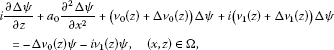(10)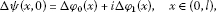(11)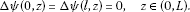(12)

Because the problem (10)-(12) and the problem (2)-(4) are the same type problems, we can write the following estimate the same as (8):

${\parallel \mathrm{\Delta }\psi \left(\mathbf{\cdot },z\right)\parallel }^{2}\le {c}_{4}{\parallel \mathrm{\Delta }\psi \parallel }_{{L}_{2}\left(0,l\right)}^{2}+{\parallel \mathrm{\Delta }{v}_{0}\psi +i\mathrm{\Delta }{v}_{1}\psi \parallel }_{{L}_{2}\left(\mathrm{\Omega }\right)}^{2},\phantom{\rule{1em}{0ex}}\mathrm{\forall }z\in \left[0,L\right].$
(13)

If we use estimate (13) then we can write the following estimate:

${\parallel \mathrm{\Delta }\psi \left(\mathbf{\cdot },z\right)\parallel }_{{L}_{2}\left(0,l\right)}^{2}\le {c}_{5}{\parallel \mathrm{\Delta }v\parallel }_{H}^{2},\phantom{\rule{1em}{0ex}}\mathrm{\forall }z\in \left[0,L\right].$
(14)

${c}_{5}>0$ is constant that does not depend on Δv.

Now, let us evaluate the increment of the functional ${J}_{0}\left(v\right)$ on $v\in V$. Using formula (9) we can write the equality as

$\begin{array}{rcl}\mathrm{\Delta }{J}_{0}\left(v\right)& =& {J}_{0}\left(v+\mathrm{\Delta }v\right)-{J}_{0}\left(v\right)\\ =& 2{\int }_{0}^{l}Re\left(\psi \left(x,L\right)-y\left(x\right)\right)\mathrm{\Delta }\overline{\psi }\left(x,L\right)\phantom{\rule{0.2em}{0ex}}dx+{\parallel \mathrm{\Delta }\psi \left(\mathbf{\cdot },L\right)\parallel }_{{L}_{2}\left(0,l\right)}^{2}.\end{array}$
(15)

Using the Cauchy-Bunyakowski inequality and estimates (8) and (14), we write the inequality as

$|\mathrm{\Delta }{J}_{0}\left(v\right)|\le {c}_{6}{\parallel \mathrm{\Delta }v\parallel }_{H}^{2},\phantom{\rule{1em}{0ex}}\mathrm{\forall }v\in V,$
(16)

where ${c}_{6}>0$ is a constant that does not depend on Δv. This inequality shows that the functional ${J}_{0}\left(v\right)$ is continuous on the set V. On the other hand, ${J}_{0}\left(z\right)\ge 0$ for $\mathrm{\forall }z\in V$; therefore, ${J}_{0}\left(v\right)$ is bounded on V. The set V is closed, bounded on a Hilbert space H. According to Theorem (Goebel) in , there is such a set G dense in H that optimalcontrol problem (1)-(4) has a unique solution for $\alpha >0$ and $\mathrm{\forall }\omega \in G$. Theorem 2 is proven. □

### 3.1 Fréchet diffrentiability of the functional

In this section, we prove the Fréchet differentiability of a given functional. For this purpose, we consider the following adjoint boundary value problem: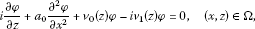(17)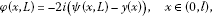(18)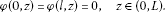(19)

Here, the function $\psi =\psi \left(x,z\right)\equiv \psi \left(x,z;v\right)$ is a solution of (2)-(4) for $v\in V$. The solution of the boundary value problem (17)-(19) corresponding to $v\in V$ is a function $\phi =\phi \left(x,z\right)$ that belongs to the space ${C}^{0}\left(\left[0,L\right],{L}_{2}\left(0,L\right)\right)$ and satisfies the integral identity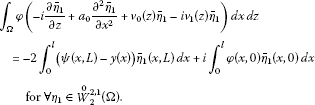(20)

As seen, the problem (17)-(19) is an initial boundary value problem. This can easily be obtained by a transform $\theta =L-z$. Actually, if we do a variable transform $\theta =L-z$, we obtain the boundary problem as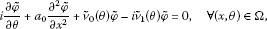(21)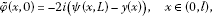(22)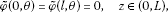(23)

where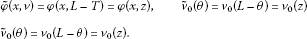If we write the complex conjugate of this boundary value problem, we obtain the following boundary value problem: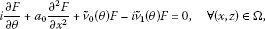(24)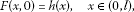(25)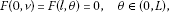(26)

where

$F\left(x,\theta \right)=\overline{\stackrel{˜}{\phi }\left(x,\theta \right)},\phantom{\rule{2em}{0ex}}h\left(x\right)=-2i\left(\overline{\psi }\left(x,L\right)-\overline{y}\left(x\right)\right).$

This problem is a type of (2)-(4) boundary value problem. As the right-hand side is equal to zero, and initial function $h\left(x\right)$ belongs to the space ${L}_{2}\left(0,l\right)$ for $\psi \in {C}^{0}\left(\left[0,L\right],{L}_{2}\left(0,l\right)\right)$, $y\in {L}_{2}\left(0,l\right)$. By using Theorem 2, it follows that the solution of the bounded value problem (24)-(26) existing in the space ${C}^{0}\left(\left[0,L\right],{L}_{2}\left(0,l\right)\right)$ is unique, and the following estimate is obtained:

${\parallel F\left(\mathbf{\cdot },\theta \right)\parallel }_{{L}_{2}\left(0,l\right)}^{2}\le {c}_{7}{\parallel h\parallel }_{{L}_{2}\left(0,l\right)}^{2},\phantom{\rule{1em}{0ex}}\mathrm{\forall }\theta \in \left[0,L\right].$
(27)

If we use the problem (24)-(26) as a type of the conjugate problem (17)-(19), we obtain the initial bounded value problem (17)-(19) has a unique solution belonging to the space ${C}^{0}\left(\left[0,L\right],{L}_{2}\left(0,l\right)\right)$, and the following estimate is obtained:

${\parallel \phi \left(\mathbf{\cdot },z\right)\parallel }_{{L}_{2}\left(0,l\right)}^{2}\le {c}_{8}{\parallel \psi \left(\mathbf{\cdot },L\right)-y\parallel }_{{L}_{2}\left(0,l\right)}^{2},\phantom{\rule{1em}{0ex}}\mathrm{\forall }z\in \left[0,L\right].$

Here, the number ${c}_{8}>0$ is independent of ψ and y. Now, using estimate (8) in this inequality, we easily write the following estimate:

${\parallel \phi \left(\mathbf{\cdot },z\right)\parallel }_{{L}_{2}\left(0,l\right)}^{2}\le {c}_{9}\left({\parallel \phi \parallel }_{{L}_{2}\left(0,l\right)}^{2}+{\parallel y\parallel }_{{L}_{2}\left(0,l\right)}^{2}+{\parallel \phi \parallel }_{{L}_{2}\left(\mathrm{\Omega }\right)}^{2}\right),\phantom{\rule{1em}{0ex}}\mathrm{\forall }z\in \left[0,L\right].$
(28)

Here, the number ${c}_{9}>0$ is constant.

Theorem 3 Let us accept that the conditions of Theorem 2 hold and $\omega \in H$ is given. Then the functional ${J}_{\alpha }\left(v\right)$ can be Frechet differentiable in the set V and the formula below for a gradient of the functional is valid: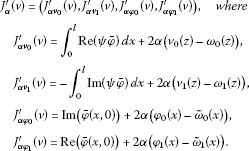(29)

Proof Let us evaluate the increment of the functional ${J}_{\alpha }\left(v\right)$ for the element $\mathrm{\forall }v\in V$. We can write the following equation for the increment of the functional:

$\begin{array}{rcl}\mathrm{\Delta }{J}_{\alpha }\left(v\right)& =& {J}_{\alpha }\left(v+\mathrm{\Delta }v\right)-{J}_{\alpha }\left(v\right)\\ =& 2{\int }_{0}^{l}Re\left[\left(\psi \left(x,L\right)-y\left(x\right)\right)\mathrm{\Delta }\overline{\psi }\left(x,L\right)\right]\phantom{\rule{0.2em}{0ex}}dx+2\alpha {\int }_{0}^{l}\left({\phi }_{0}\left(x\right)-{\stackrel{˜}{\omega }}_{0}\left(x\right)\right)\mathrm{\Delta }{\phi }_{0}\left(x\right)\phantom{\rule{0.2em}{0ex}}dx\\ +2\alpha {\int }_{0}^{l}\left({\phi }_{1}\left(x\right)-{\stackrel{˜}{\omega }}_{1}\left(x\right)\right)\mathrm{\Delta }{\phi }_{1}\left(x\right)\phantom{\rule{0.2em}{0ex}}dx+2\alpha {\int }_{0}^{T}\left({v}_{0}\left(z\right)-{\omega }_{0}\left(z\right)\right)\mathrm{\Delta }{v}_{0}\left(z\right)\phantom{\rule{0.2em}{0ex}}dz\\ +2\alpha {\int }_{0}^{T}\left({v}_{1}\left(z\right)-{\omega }_{1}\left(z\right)\right)\mathrm{\Delta }{v}_{1}\left(z\right)\phantom{\rule{0.2em}{0ex}}dz+{\parallel \mathrm{\Delta }\psi \left(\mathbf{\cdot },L\right)\parallel }_{{L}_{2}\left(0,l\right)}^{2}+\alpha {\parallel \mathrm{\Delta }v\parallel }_{H}^{2}.\end{array}$
(30)

The last formula can be written as follows:

$\begin{array}{rcl}\mathrm{\Delta }{J}_{\alpha }\left(v\right)& =& {J}_{\alpha }\left(v+\mathrm{\Delta }v\right)-{J}_{\alpha }\left(v\right)\\ =& {\int }_{0}^{L}\left({\int }_{0}^{l}Re\left(\psi \overline{\phi }\right)\phantom{\rule{0.2em}{0ex}}dx+2\alpha \left({v}_{0}\left(z\right)-{\omega }_{0}\left(z\right)\right)\mathrm{\Delta }{v}_{0}\left(z\right)\phantom{\rule{0.2em}{0ex}}dz-{\int }_{0}^{l}Im\left(\psi ,\overline{\phi }\right)\phantom{\rule{0.2em}{0ex}}dx\mathrm{\Delta }{v}_{1}\left(z\right)\right)\\ +{\int }_{0}^{L}\left(-{\int }_{0}^{l}Im\left(\psi \overline{\phi }\right)\phantom{\rule{0.2em}{0ex}}dx+2\alpha \left({v}_{1}\left(z\right)-{\omega }_{1}\left(z\right)\right)\right)\mathrm{\Delta }{v}_{1}\left(z\right)\phantom{\rule{0.2em}{0ex}}dz\\ +{\int }_{0}^{l}\left[Im\left(\overline{\phi }\left(x,0\right)\right)+2\alpha \left({\phi }_{0}\left(x\right)-{\stackrel{˜}{\omega }}_{0}\left(x\right)\right)\right]\mathrm{\Delta }{\phi }_{0}\left(x\right)\phantom{\rule{0.2em}{0ex}}dx\\ +{\int }_{0}^{l}\left[Re\left(\overline{\phi }\left(x,0\right)\right)+2\alpha \left({\phi }_{1}\left(x\right)-{\stackrel{˜}{\omega }}_{1}\left(x\right)\right)\right]\mathrm{\Delta }{\phi }_{1}\left(x\right)\phantom{\rule{0.2em}{0ex}}dx+R\left(\mathrm{\Delta }v\right),\end{array}$

where $R\left(\mathrm{\Delta }v\right)$ is defined as the formula

$\begin{array}{rcl}R\left(\mathrm{\Delta }v\right)& =& {\parallel \mathrm{\Delta }\psi \left(\mathbf{\cdot },L\right)\parallel }_{{L}_{2}\left(0,l\right)}^{2}+\alpha {\parallel \mathrm{\Delta }v\parallel }_{H}^{2}\\ +{\int }_{\mathrm{\Omega }}Re\left(\mathrm{\Delta }\psi \overline{\phi }\right)\mathrm{\Delta }{v}_{0}\left(z\right)\phantom{\rule{0.2em}{0ex}}dx\phantom{\rule{0.2em}{0ex}}dz\\ -{\int }_{\mathrm{\Omega }}Im\left(\mathrm{\Delta }\psi \overline{\phi }\right)\mathrm{\Delta }{v}_{1}\left(z\right)\phantom{\rule{0.2em}{0ex}}dx\phantom{\rule{0.2em}{0ex}}dz.\end{array}$
(31)

Applying the Cauchy-Bunyakowski inequality, we obtain:

$\begin{array}{rcl}|R\left(\mathrm{\Delta }v\right)|& \le & {\parallel \mathrm{\Delta }\psi \left(\mathbf{\cdot },L\right)\parallel }_{{L}_{2}\left(0,l\right)}^{2}+\alpha {\parallel \mathrm{\Delta }v\parallel }_{H}^{2}\\ +\left({\parallel \mathrm{\Delta }{v}_{1}\parallel }_{{L}_{2}\left(0,T\right)}+{\parallel \mathrm{\Delta }{v}_{0}\parallel }_{{L}_{2}\left(0,T\right)}\right)max{\parallel \mathrm{\Delta }\psi \left(\mathbf{\cdot },L\right)\parallel }_{{L}_{2}\left(0,l\right)}{\parallel \phi \parallel }_{{L}_{2}\left(0,L\right)}.\end{array}$

If we use estimates (13) and (28) in this inequality, we obtain

$|R\left(\mathrm{\Delta }v\right)|\le {c}_{10}{\parallel \mathrm{\Delta }v\parallel }_{H}^{2}.$
(32)

Here, ${c}_{10}>0$ is a constant that does not depend on Δv. Hence, we write

$R\left(\mathrm{\Delta }v\right)=o\left({\parallel \mathrm{\Delta }v\parallel }_{H}\right).$
(33)

By using equality (33), the increment of the functional can be written as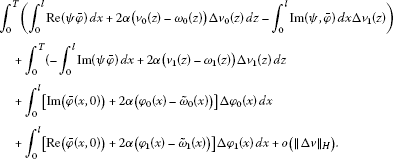(34)

Considering this equality (34), and by using the definition of Fréchet differentiable, we can easily obtain the validity of the rule. Theorem 3 is proved. □

### 3.2 A necessary condition for an optimal solution

In this section, we prove the continuity of a gradient and state a necessary condition to an optimal solution in the variational inequality form using the gradient.

Theorem 4 Accept that the conditions of Theorem 3 hold and ${v}^{\ast }\in V$ is an optimal solution of the problem (1)-(4). Then the following inequality is valid for $\mathrm{\forall }v\in V$: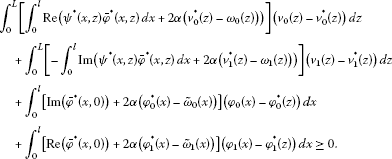(35)

Here, the functions ${\psi }^{\ast }\left(x,z\right)\equiv \psi \left(x,z;{v}^{\ast }\right)$, ${\phi }^{\ast }\left(x,z\right)\equiv \phi \left(x,z;{v}^{\ast }\right)$ are solutions of the problems (2)-(4) and a conjugate problem corresponding to ${v}^{\ast }\in V$, respectively.

Proof Now, we prove that the gradient ${J}_{\alpha }^{\prime }\left(v\right)$ is continuous at V. For this we show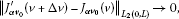(36)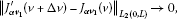(37)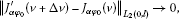(38)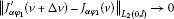(39)

for ${\parallel \mathrm{\Delta }v\parallel }_{H}\to 0$.

In order to show (36), using the formula ${J}_{\alpha {v}_{0}}^{\prime }\left(v\right)={\int }_{0}^{l}Re\left(\psi \overline{\phi }\right)\phantom{\rule{0.2em}{0ex}}dx+2\alpha \left({v}_{0}\left(z\right)-{\omega }_{0}\left(z\right)\right)$ in (29), we can write the following equation: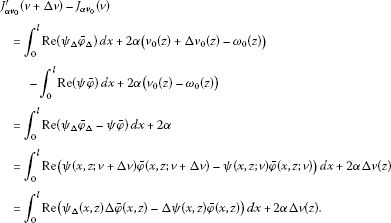(40)

Here, $\mathrm{\Delta }\psi =\mathrm{\Delta }\psi \left(x,z\right)$ is the solution of the problem (9)-(11) and $\mathrm{\Delta }\phi =\mathrm{\Delta }\phi \left(x,z\right)$ is the solution of the following problem: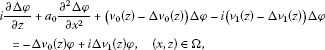(41)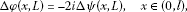(42)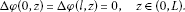(43)

This bounded value problem is a type of a conjugate problem. For this solution, the following estimate is valid:

${\parallel \phi \left(\mathbf{\cdot },z\right)\parallel }_{{L}_{2}\left(0,l\right)}^{2}\le {c}_{11}\left({\parallel \mathrm{\Delta }{v}_{0}\phi +i\mathrm{\Delta }{v}_{0}\phi \parallel }_{{L}_{2}\left(\mathrm{\Omega }\right)}^{2}+{\parallel \mathrm{\Delta }\psi \left(\mathbf{\cdot },L\right)\parallel }_{{L}_{2}\left(0,l\right)}^{2}\right),\phantom{\rule{1em}{0ex}}\mathrm{\forall }z\in \left(0,L\right).$
(44)

Here, the number ${c}_{11}$ is constant.

Using (13) and (28), we write

${\parallel \phi \left(\mathbf{\cdot },z\right)\parallel }_{{L}_{2}\left(0,l\right)}^{2}\le {c}_{12}\left({\parallel \mathrm{\Delta }v\parallel }_{H}^{2}\right),\phantom{\rule{1em}{0ex}}\mathrm{\forall }z\in \left(0,L\right).$
(45)

Here, the number ${c}_{12}$ is constant. Using (13) and (45) and applying the Cauchy-Bunyakovski inequality, we obtain

$\begin{array}{rcl}|{J}_{\alpha {v}_{0}}^{\prime }\left(v+\mathrm{\Delta }v\right)-{J}_{\alpha {v}_{0}}^{\prime }\left(v\right)|& \le & {\parallel {\psi }_{\mathrm{\Delta }}\left(\mathbf{\cdot },z\right)\parallel }_{{L}_{2}\left(0,l\right)}{\parallel \mathrm{\Delta }\phi \left(\mathbf{\cdot },z\right)\parallel }_{{L}_{2}\left(0,l\right)}\\ +{\parallel \mathrm{\Delta }\psi \left(\mathbf{\cdot },z\right)\parallel }_{{L}_{2}\left(0,l\right)}{\parallel \phi \left(\mathbf{\cdot },z\right)\parallel }_{{L}_{2}\left(0,l\right)}+2\alpha |\mathrm{\Delta }{v}_{0}\left(z\right)|,\phantom{\rule{1em}{0ex}}\mathrm{\forall }z\in \left(0,L\right),\end{array}$

and then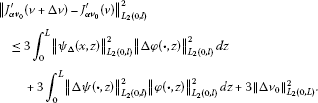(46)

If we use estimate (8), we can write the following inequality:

${\parallel {\psi }_{\mathrm{\Delta }}\left(\mathbf{\cdot },z\right)\parallel }_{{L}_{2}\left(0,l\right)}^{2}\le {c}_{13},\phantom{\rule{1em}{0ex}}\mathrm{\forall }z\in \left[0,L\right].$
(47)

Using this inequality and estimates (13), (28), and (45), we obtain

${\parallel {J}_{\alpha {v}_{0}}^{\prime }\left(v+\mathrm{\Delta }v\right)-{J}_{\alpha {v}_{0}}^{\prime }\left(v\right)\parallel }_{{L}_{2}\left(0,L\right)}\le {c}_{14}{\parallel \mathrm{\Delta }v\parallel }_{H}.$
(48)

Here, the number of ${c}_{14}$ is constant. Similarly, we can prove the following inequality:

${\parallel {J}_{\alpha {v}_{1}}^{\prime }\left(v+\mathrm{\Delta }v\right)-{J}_{\alpha {v}_{1}}^{\prime }\left(v\right)\parallel }_{{L}_{2}\left(0,L\right)}\le {c}_{15}{\parallel \mathrm{\Delta }v\parallel }_{H}.$
(49)

If we use inequalities (48) and (49), we see that the correlations limit (36) and (37) is valid.

Now, we prove (38). To prove this using the formula ${J}_{\alpha {\phi }_{1}}^{\prime }\left(v\right)=Re\left(\overline{\phi }\left(x,0\right)\right)+2\alpha \left({\phi }_{1}\left(x\right)-{\stackrel{˜}{\omega }}_{1}\left(x\right)\right)$ in (29), we can write the following inequality:

${J}_{\alpha {v}_{0}}^{\prime }\left(v+\mathrm{\Delta }v\right)-{J}_{\alpha {v}_{0}}^{\prime }\left(v\right)={I}_{m}\left(\mathrm{\Delta }\overline{\phi }\left(x,0\right)\right)+2\alpha \mathrm{\Delta }{\phi }_{0}.$
(50)

Here, $\mathrm{\Delta }\phi \left(x,z\right)$ is a solution of the problem (41). Estimate (45) is valid for $\mathrm{\forall }z\in \left[0,L\right]$. Therefore, the following estimate can be written at $z=0$:

${\parallel \phi \left(\mathbf{\cdot },0\right)\parallel }_{{L}_{2}\left(0,l\right)}^{2}\le {c}_{12}\left({\parallel \mathrm{\Delta }v\parallel }_{H}^{2}\right).$

If this inequality is used in (49), we easily can write

${\parallel {J}_{\alpha {\phi }_{0}}^{\prime }\left(v+\mathrm{\Delta }v\right)-{J}_{\alpha {\phi }_{0}}^{\prime }\left(v\right)\parallel }_{{L}_{2}\left(0,l\right)}\le {c}_{16}\left({\parallel \mathrm{\Delta }v\parallel }_{H}\right).$
(51)

Similarly, if we use (39), we obtain

${\parallel {J}_{\alpha {\phi }_{1}}^{\prime }\left(v+\mathrm{\Delta }v\right)-{J}_{\alpha {\phi }_{1}}^{\prime }\left(v\right)\parallel }_{{L}_{2}\left(0,l\right)}\le {c}_{17}\left({\parallel \mathrm{\Delta }v\parallel }_{H}\right).$
(52)

We can see that (38) and (39) are valid by inequalities (51) and (52). That is, ${J}_{\alpha }\in {C}^{1}\left(V\right)$. On the other hand, V is a convex set according to the definition. So, the functional ${J}_{\alpha }\left(v\right)$ holds by the condition of Theorem (Goebel) in  at V. Therefore, considering Theorem 3, we obtain

${〈{J}_{\alpha }^{\prime }\left({v}^{\ast }\right),v-{v}^{\ast }\right)〉}_{H}\ge 0$

for $\mathrm{\forall }z\in V$. Here, using (29), it is seen that the statement of Theorem 4 is valid. □

## References

1. İskenderov AD, Yagubov GY: Optimal control of non-linear quantum-mechanical systems. Autom. Remote Control 1989, 50: 1631-1641.

2. İskenderov AD, Yagubov GY: A variational method for solving the inverse problem of determining the quantumnmechanical potential. Sov. Math. Doklady (Engl. Trans.) Am. Math. Soc. 1989, 38: 637-641.

3. Yagubov GY, Musayeva MA: About the problem of identification for nonlinear Schrödinger equation. J. Differ. Equ. 1997, 33(12):1691-1698.

4. Yagubov, GY: Optimal control by coefficient of quasilinear Schrödinger equation. Abstract of these doctors sciences, Kiev, p. 25 (1994)

5. Yetişkin H, Subaşı M: On the optimal control problem for Schrödinger equation with complex potential. Appl. Math. Comput. 2010, 216: 1896-1902. 10.1016/j.amc.2010.03.039

6. Yıldız B, Yagubov G: On the optimal control problem. J. Comput. Appl. Math. 1997, 88: 275-287. 10.1016/S0096-3003(96)00335-9

7. Ladyzhenskaya OA, Solonnikov VA, Uralsteva NN Translation of Mathematical Monograps. In Linear and Quasi-Linear Equations of Parabolic Type. Am. Math. Soc., Providence; 1968.

8. Goebel M: On existence of optimal control. Math. Nachr. 1979, 93: 67-73. 10.1002/mana.19790930106

## Author information

Authors

### Corresponding author

Correspondence to Yusuf Koçak.

### Competing interests

The authors declare that they have no competing interests.

### Authors’ contributions

YK carried out the optimal control problem studies, participated in the sequence alignment and drafted the manuscript. EÇ conceived of the study and, participated in its design and coordination. All authors read and approved the final manuscript.

## Rights and permissions

Reprints and Permissions

Koçak, Y., Çelik, E. Optimal control problem for stationary quasi-optic equations. Bound Value Probl 2012, 151 (2012). https://doi.org/10.1186/1687-2770-2012-151

• Accepted:

• Published:

• DOI: https://doi.org/10.1186/1687-2770-2012-151

### Keywords

• stationary equation of quasi optic
• boundary value problem
• optimal control problem
• variational problem